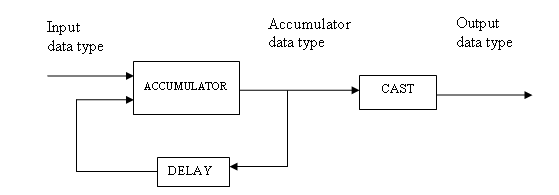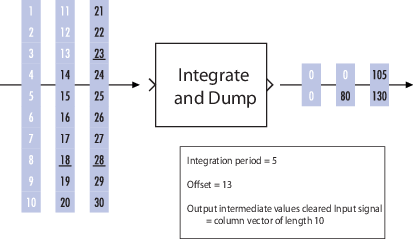# Integrate and Dump

Integrate discrete-time signal, resetting to zero periodically

## Library

Comm Filters

•## Description

The Integrate and Dump block creates a cumulative sum of the discrete-time input signal, while resetting the sum to zero according to a fixed schedule. When the simulation begins, the block discards the number of samples specified in the Offset parameter. After this initial period, the block sums the input signal along columns and resets the sum to zero every N input samples, where N is the Integration period parameter value. The reset occurs after the block produces its output at that time step.

Receiver models often use the integrate-and-dump operation when the system's transmitter uses a simple square-pulse model. Fiber optics and in spread-spectrum communication systems, such as CDMA (code division multiple access) applications, also use the operation.

This block accepts a scalar, column vector, or matrix input signal. When the input signal is not a scalar value, it must contain k·N rows for some positive integer k. For these input signals, the block processes each column independently.

Selecting Output intermediate values affects the contents, dimensions, and sample time as follows:

• If you clear the check box, then the block outputs the cumulative sum at each reset time.

• If the input is a scalar value, then the output sample time is N times the input sample time and the block experiences a delay whose duration is one output sample period. In this case, the output dimensions match the input dimensions.

• If the input is a (k·N)-by-n matrix, then the output is k-by-n. In this case, the block experiences no delay and the output period matches the input period.

• If you select the check box, then the block outputs the cumulative sum at each time step. The output has the same sample time and the same matrix dimensions as the input.

### Transients and Delays

A nonzero value in the Offset parameter causes the block to output one or more zeros during the initial period while it discards input samples. If the input is a matrix with n columns and the Offset parameter is a length-n vector, then the mth element of the Offset vector is the offset for the mth column of data. If Offset is a scalar, then the block applies the same offset to each column of data. The output of initial zeros due to a nonzero Offset value is a transient effect, not a persistent delay.

When you clear Output intermediate values, the block's output is delayed, relative to its input, throughout the simulation:

• If the input is a scalar value, then the output is delayed by one sample after any transient effect is over. That is, after removing transients from the input and output, you can see the result of the mth integration period in the output sample indexed by m+1.

• If the input is a column vector or matrix and the Offset parameter is nonzero, then after the transient effect is over, the result of each integration period appears in the output frame corresponding to the last input sample of that integration period. This is one frame later than the output frame corresponding to the first input sample of that integration period, in cases where an integration period spans two input frames. For an example of this situation, see Example of Transient and Delay.

## Parameters

Integration period

The number of input samples between resets.

Offset

A nonnegative integer vector or scalar specifying the number of input samples to discard from each column of input data at the beginning of the simulation.

Output intermediate values

Determines whether the block outputs the intermediate cumulative sums between successive resets.

### Fixed-Point Signal Flow Diagram### Fixed-Point Attributes

The settings for the following parameters only apply when block inputs are fixed-point signals.

Rounding mode

Use this parameter to specify the rounding method to be used when the result of a fixed-point calculation does not map exactly to a number representable by the data type and scaling storing the result.

For more information, see Rounding Modes or Rounding Mode: Simplest (Fixed-Point Designer).

Saturate on integer overflow

Use this parameter to specify the method to be used if the magnitude of a fixed-point calculation result does not fit into the range of the data type and scaling that stores the result:

• Saturate represents positive overflows as the largest positive number in the range being used, and negative overflows as the largest negative number in the range being used.

• Wrap uses modulo arithmetic to cast an overflow back into the representable range of the data type. See Modulo Arithmetic (Fixed-Point Designer) for more information.

Accumulator—Mode

Use the Accumulator—Mode parameter to specify how you would like to designate the accumulator word and fraction lengths:

• When you select ```Inherit via internal rule```, the block automatically calculates the accumulator output word and fraction lengths.

• When you select `Same as input`, these characteristics match those of the input to the block.

• When you select ```Binary point scaling```, you are able to enter the word length and the fraction length of the accumulator, in bits.

• When you select ```Slope and bias scaling```, you are able to enter the word length, in bits, and the slope of the accumulator.

Output

Use the Output parameter to choose how you specify the word length and fraction length of the output of the block:

• When you select ```Same as accumulator```, these characteristics match those of the accumulator.

• When you select `Same as input`, these characteristics match those of the input to the block.

• When you select ```Binary point scaling```, enter the word length and the fraction length of the output, in bits.

• When you select ```Slope and bias scaling```, enter the word length, in bits, and the slope of the output.

For additional information about the parameters pertaining to fixed-point applications, see Specify Fixed-Point Attributes for Blocks.

## Supported Data Type

PortSupported Data Types
In
• Double-precision floating point

• Single-precision floating point

• Fixed-point

Out
• Double-precision floating point

• Single-precision floating point

• Fixed-point

## Examples

If Integration period is `4` and Offset is the scalar `3`, then the table below shows how the block treats the beginning of a ramp (`1`, `2`, `3`, `4`,...) in several situations. (The values shown in the table do not reflect vector sizes but merely indicate numerical values.)

Output intermediate values Check BoxInput Signal PropertiesFirst Several Output Values
Cleared Scalar `0`, `0`, `4+5+6+7`, and `8+9+10+11`, where one `0` is an initial transient value and the other `0` is a delay value that results from the cleared check box and scalar value input.
Cleared Column vector of length `4` `0`, `4+5+6+7`, and `8+9+10+11`, where `0` is an initial delay value that results from the nonzero offset. The output is a scalar value.
Selected Scalar `0`, `0`, `0`, `4`, `4+5`, `4+5+6`, `4+5+6+7`, `8`, `8+9`, `8+9+10`, `8+9+10+11`, and `12`, where the three `0`s are initial transient values.
Selected Column vector of length `4` `0`, `0`, `0`, `4`, `4+5`, `4+5+6`, `4+5+6+7`, `8`, `8+9`, `8+9+10`, `8+9+10+11`, and `12`, where the three `0`s are initial transient values. The output is a column vector of length `4`.

In all cases, the block discards the first three input samples (`1`, `2`, and `3`).

### Example of Transient and Delay

The figure below illustrates a situation in which the block exhibits both a transient effect for three output samples, as well as a one-sample delay in alternate subsequent output samples for the rest of the simulation. The figure also indicates how the input and output values are organized as column vectors. In each vector in the figure, the last sample of each integration period is underlined, discarded input samples are white, and transient zeros in the output are white.The transient effect lasts for `ceil(13/5)` output samples because the block discards `13` input samples and the integration period is `5`. The first output sample after the transient effect is over, `80`, corresponds to the sum `14+15+16+17+18` and appears at the time of the input sample `18`. The next output sample, `105`, corresponds to the sum `19+20+21+22+23` and appears at the time of the input sample `23`. Notice that the input sample `23` is one frame later than the input sample `19`; that is, this five-sample integration period spans two input frames. As a result, the output of `105` is delayed compared to the first input (`19`) that contributes to that sum.